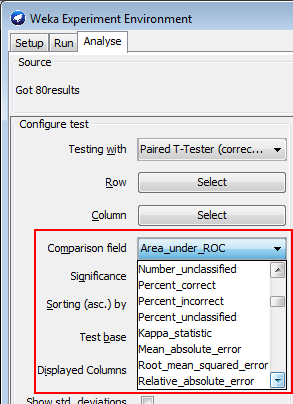# Statistics - Hypothesis (Tests|Testing)

Hypothesis testing is a test of a certain value of a parameter.

The most common hypothesis test involves testing the null hypothesis (ie the parameter is Null)

The output of a test is binary: true or false.

Recommended PagesData Mining - (Parameters | Model) (Accuracy | Precision | Fit | Performance) Metrics

Accuracy is a evaluation metrics on how a model perform. rare event detection Hypothesis testing: t-statistic and p-value. The p value and t statistic measure how strong is the...Statistics - (Student's) t-test (Mean Comparison)

The t-test is a test that compares means. NHST can be conducted yielding to a p-value Effect Size can be calculated like in multiple regression. Confidence Interval around the mean can also be...Statistics - (t-value|t-statistic)

The (t-value|t-statistic) is a test statistic. In NHST, it is essentially a ratio of what we observed relative to what we would expect just due to chance. Each t-value has corresponding p-value depending...Statistics - A/B (Test|Testing)

A/B Null Hypothesis test set up multiple copies of the website, redirect users randomly to each copies via 307 status measure which design improves the target the most. Gather Samples Assign...Statistics - Confidence Interval

The definition of a confidence interval says that under repeated experiments 95% of the time this confidence interval will contain the true statistic (mean, ...). if we started the whole experiment over...Statistics - Null Hypothesis Significance Testing (NHST)

NHST is a procedure for testing the Null Hypothesis. It's a binary decision: Reject or Retain the Null Hypothesis Ie Retain the Null Hypothesis or the alternative hypothesis. Before starting any...Statistics - Post-hoc test

In an anova, the Post-hoc tests is used to figure out exactly where there are significant differences.Statistics - Power of a test

The power of a test sometimes, less formally, refers to the probability of rejecting the null when it is not correct, the chance that your experiment is right. A test's power is influenced by the choice...Statistics - Statistic

In general, each statistic is an estimate of a parameter, whose value is not known exactly. Every number found using a sample is just an (approximation|estimation) of a parameter (the truth). in descriptive...Test

How to unit test machine learning code